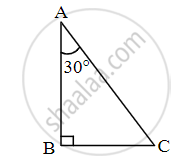# From given figure, In ∆ABC, If ∠ABC = 90° ∠CAB=30°, AC = 14 then for finding value of AB and BC, complete the following activity.Activity: In ∆ABC, If ∠ABC = 90° ∠CAB=30° ∴ ∠BCA = □ By theorem of 30° - Geometry

Sum

From given figure, In ∆ABC, If ∠ABC = 90° ∠CAB=30°, AC = 14 then for finding value of AB and BC, complete the following activity.Activity: In ∆ABC, If ∠ABC = 90°, ∠CAB=30°

∴ ∠BCA = square

By theorem of 30° – 60° – 90° triangle,

∴ square = 1/2 AC and square = sqrt(3)/2 AC

∴ BC = 1/2 xx square and AB = sqrt(3)/2 xx 14

∴ BC = 7 and AB = 7sqrt(3)

#### Solution

In ∆ABC, If ∠ABC = 90°, ∠CAB=30°

∴ ∠BCA = 60°     ......[Remaining angle of a triangle]

By theorem of 30° – 60° – 90° triangle,

∴ BC = 1/2 AC      .....[Side opposite to 30°]

and AB = sqrt(3)/2 AC   .....[Side opposite to 60°]

∴ BC = 1/2 xx 14 and AB = sqrt(3)/2 xx 14

∴ BC = 7 and AB = 7sqrt(3)

Concept: Property of 30°- 60°- 90° Triangle Theorem
Is there an error in this question or solution?